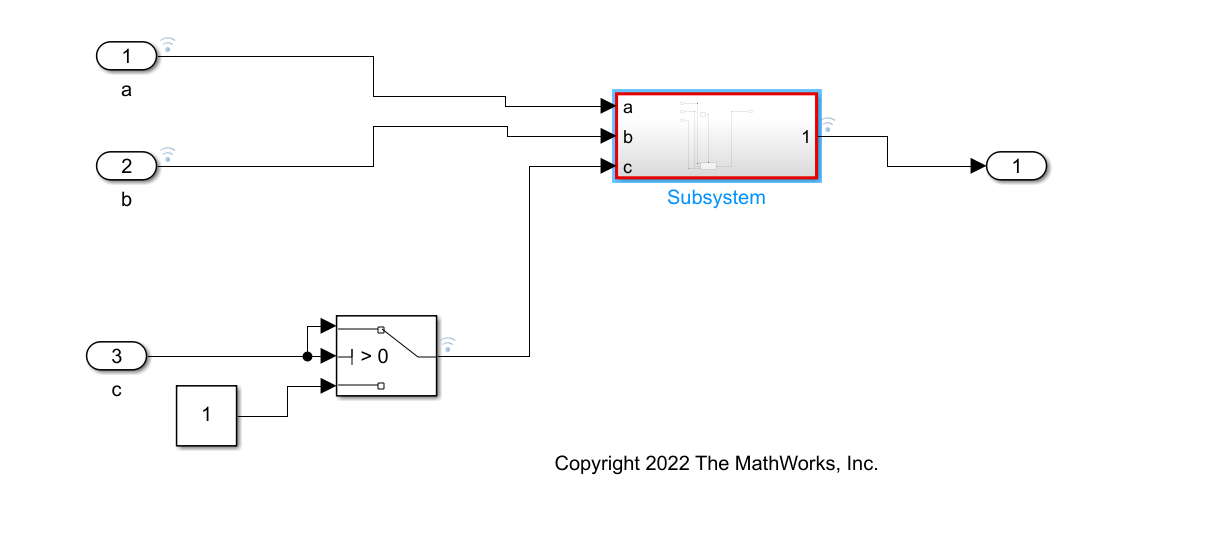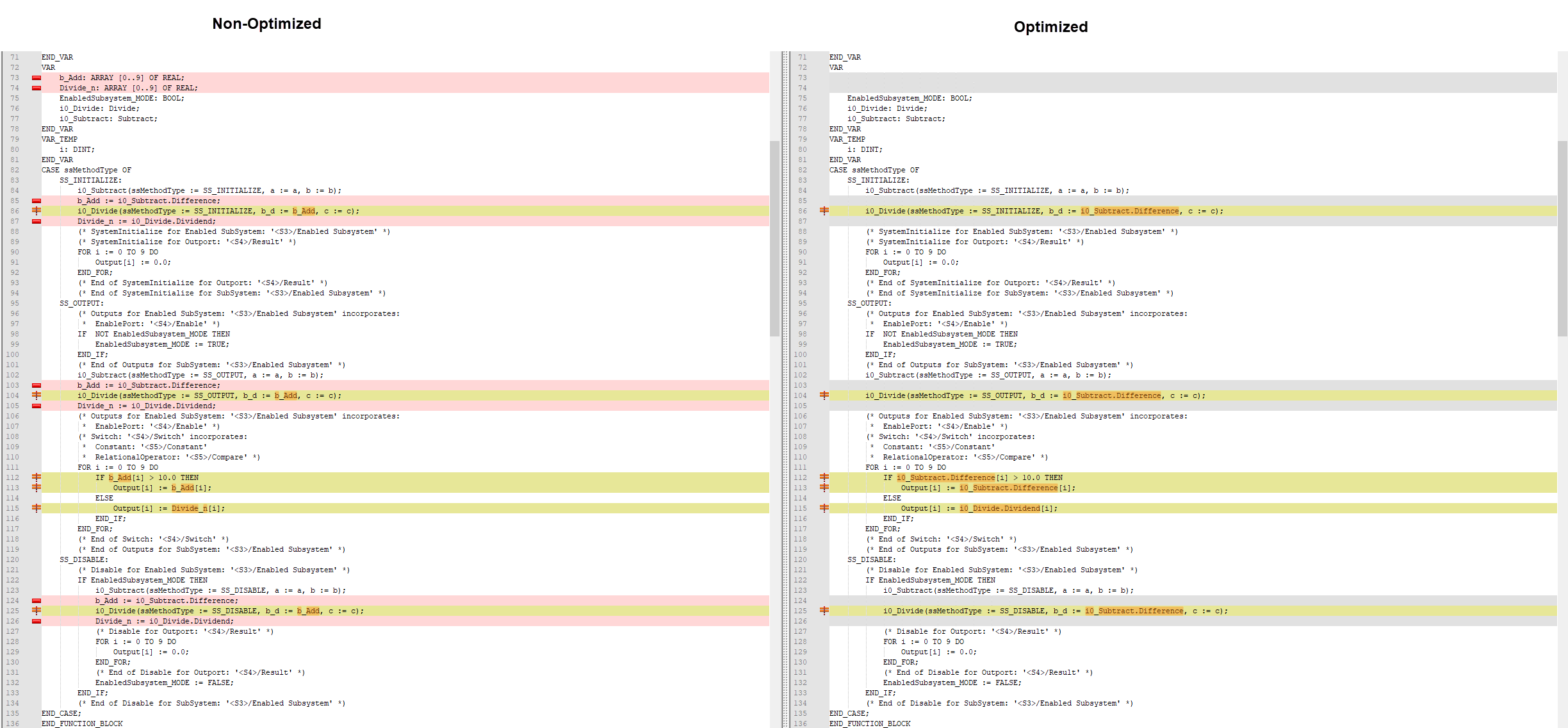# Generate Optimized Structured Text Code by Folding Expressions

This example shows how to generate optimized structured text code by optimizing the output variables of function blocks generated from reusable Simulink® subsystems. Optimize the output variables by using expression folding to minimize the computation of intermediate results at block outputs and the storage of these results in temporary buffers or variables. When you enable the Fold temporary variable using Function block instance output variable optimization, Simulink® PLC Coder™ folds the function block computations into a single expression, instead of generating separate code statements and storage declarations for each function block.

### Open the Model

`open_system('Calculator.slx')`

The model consists of a subsystem at the top level that accepts the inputs `a`, `b`, and `c`. The subsystem contains a reusable masked subsystem, `fb_Calculate`, that houses an enabled subsystem consisting of:

• `fb_Subtract `— A reusable function block that subtracts `b` from `a`.

• `fb_Divide` — A reusable function block that divides the output of `fb_Subtract` by `c`.

The output of the subsystem is a switched output signal that outputs the result of `fb_Subtract` if the difference is greater than ten and the output of `fb_Divide` when the difference is less than ten.### Enable Optimization

To enable the Fold temporary variable using function block instance output variable optimization using the Simulink® PLC Coder™ app:

1. In the apps tab, click PLC Coder.

2. In the PLC Coder tab, click Settings > PLC Code Generation Settings. In the Configuration Parameters window, click Optimizations.

3. Select Fold temporary variable using Function Block instance output variable.

4. Click OK.

Alternatively, to enable the optimization by using the MATLAB® command line, enter:

```set_param('Calculator','PLC_FoldFBCallOutputVar','on') ```

### Generate Structured Text Code

To generate structured text code using the Simulink® PLC Coder™ app:

1. In the PLC Coder tab, click Settings > PLC Code Generation Settings. Change the Target IDE to `3S CodeSys 2.3`. Click OK.

2. Select the Subsystem block. In the PLC Code tab, click Generate PLC Code.

Alternatively, to generate structured text code from the MATLAB command line, use the `plcgeneratecode` function.

`generatedfiles = plcgeneratecode('Calculator/Subsystem');`
```### Generating PLC code for 'Calculator/Subsystem'. ### Using model settings from 'Calculator' for PLC code generation parameters. ### Gathering test vectors for PLC testbench. ### Begin code generation for IDE codesys23. ### Emit PLC code to file. ### PLC code generation successful for 'Calculator/Subsystem'. ### Generated files: tb\Calculator.exp ```

### Non-Optimized Code and Optimized Code

This image shows the comparison between the non-optimized code where expression folding is disabled and optimized code where expression folding is enabled.When you enable the optimization, Simulink® PLC Coder™:

• Removes temporary variables and buffer declarations.

• Folds the function block output expressions into a single line. For example, `i0_Divide(ssMethodType :=SS_INITIALIZE b_d :=i0_Subtract.Difference, c :=c)`

• Replaces temporary variable calls with folded expression calls.